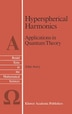Hyperspherical Harmonics: Applications in Quantum Theory

byJohn S. Avery

Pricing and Purchase Info

\$270.29 online
\$283.95 list price
Earn 1,351 plum® points

Prices and offers may vary in store

Quantity:

In stock online

Ships free on orders over \$25

Not available in stores

where d 3 3)2 ( L x - -- i3x j3x j i i>j Thus the Gegenbauer polynomials play a role in the theory of hyper spherical harmonics which is analogous to the role played by Legendre polynomials in the familiar theory of 3-dimensional spherical harmonics; and when d = 3, the Gegenbauer polynomials reduce to Legendre polynomials. The familiar sum rule, in 'lrlhich a sum of spherical harmonics is expressed as a Legendre polynomial, also has a d-dimensional generalization, in which a sum of hyper spherical harmonics is expressed as a Gegenbauer polynomial (equation (3-27»: The hyper spherical harmonics which appear in this sum rule are eigenfunctions of the generalized angular monentum 2 operator A , chosen in such a way as to fulfil the orthonormality relation: VIe are all familiar with the fact that a plane wave can be expanded in terms of spherical Bessel functions and either Legendre polynomials or spherical harmonics in a 3-dimensional space. Similarly, one finds that a d-dimensional plane wave can be expanded in terms of HYPERSPHERICAL HARMONICS xii "hyperspherical Bessel functions" and either Gegenbauer polynomials or else hyperspherical harmonics (equations ( 4 - 27) and ( 4 - 30) ) : 00 ik-x e = (d-4)!!A<_29_ 00="" _28_d-229_21_21_i28_029_="" _23a_=""><_28_kr29_><_20_28_5b_22_2k29_y20_28_5b_22_229_20_a20_a3d_o20_29_.20_l29_.20_l29_j20_where20_i28_o29_20_is20_the20_total20_solid20_angle.20_this20_expansion20_of20_a20_d-dimensional20_plane20_wave20_is20_useful20_when20_we20_wish20_to20_calculate20_fourier20_transforms20_in20_a20_d-dimensional20_space. _28_5b_22_2k29_y="" _28_5b_22_229_="" a="" _29_.="" _l29_.="" _l29_j="" where="" _i28_o29_="" is="" the="" total="" solid="" angle.="" this="" expansion="" of="" d-dimensional="" plane="" wave="" useful="" when="" we="" wish="" to="" calculate="" fourier="" transforms="" in="">
Title:Hyperspherical Harmonics: Applications in Quantum TheoryFormat:PaperbackDimensions:256 pagesPublished:January 21, 2012Publisher:Springer-Verlag/Sci-Tech/TradeLanguage:English

The following ISBNs are associated with this title:

ISBN - 10:9401075441

ISBN - 13:9789401075442